Courses

# Frequency Domain Analysis of Control Systems - 2

## 20 Questions MCQ Test Topicwise Question Bank for Electrical Engineering | Frequency Domain Analysis of Control Systems - 2

Description
This mock test of Frequency Domain Analysis of Control Systems - 2 for Electrical Engineering (EE) helps you for every Electrical Engineering (EE) entrance exam. This contains 20 Multiple Choice Questions for Electrical Engineering (EE) Frequency Domain Analysis of Control Systems - 2 (mcq) to study with solutions a complete question bank. The solved questions answers in this Frequency Domain Analysis of Control Systems - 2 quiz give you a good mix of easy questions and tough questions. Electrical Engineering (EE) students definitely take this Frequency Domain Analysis of Control Systems - 2 exercise for a better result in the exam. You can find other Frequency Domain Analysis of Control Systems - 2 extra questions, long questions & short questions for Electrical Engineering (EE) on EduRev as well by searching above.
QUESTION: 1

### If the bandwidth of the system is increased, then

Solution:

We know that,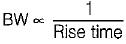Thus, if BW is increased, rise time will decrease and the system response will become fast. Due to faster response of system, damping ratio will decrease.

QUESTION: 2

### Polar plot of G(s) = 1/(1+Ts) is a

Solution:

Given, G(s) = 1/(1+Ts)
It is a type-0 and order-1 system, therefore its polar-plot will be as shown below.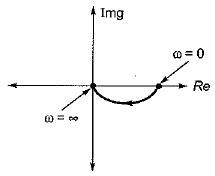Hence, the polar plot will be semicircular in shape.

QUESTION: 3

### Consider the following statements related to frequency domain analysis: 1. The cut-off rate is the slope of the log magnitude curve near the cut-off frequency. 2. The bandwidth is defined as the band of frequencies lying between 3 dB points. 3. Higher the value of resonant frequency of the system, slower is the time response. 4. The magnitude of resonant peak gives the information about the relative stability of the system. Which of these statements is/are not correct?

Solution:

The bandwidth is defined as the band of frequencies tying between-3 dB points. Hence, statement-2 is false.
Higher the value of resonant frequency of the system, faster is the time response. Hence, statement-3 is false.

QUESTION: 4

The inverse polar plot of the open loop transfer function, G(s) = (1+sT)/(sT) will be re presented by

Solution:

Given, G(s) = (1+sT)/(sT)
The inverse polar plot of G(jω) is the polar plot of 1/G(jω)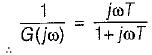Thus,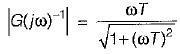and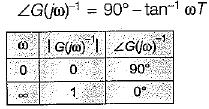Hence, inverse polar plot will be as show below,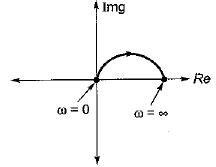QUESTION: 5

The constant M-circles corresponding to the magnitude (M) of the closed loop transfer function of a linear system for value of M less than one lie in the G-plane and to the

Solution:
QUESTION: 6

The slope of the line due to 1/(jω)factor in magnitude part of Bode plot is

Solution:

1/(jω)2 represents type-2 factor which will have a slope of -40 db/decade.
We know that,
-20 db/decase = -6 db/octave
∴ -40 db/decase = -12 db/octave

QUESTION: 7

The magnitude plot of the open loop transfer function G(s) of a certain system is shown in figure below.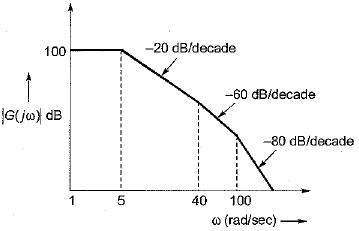If the system is of minimum phase type, then the open-loop transfer function G(s) will be given by:

Solution:

Since, the system is of minimum phase type, it has no poles or zeros in the right hand side of s-plane.
⇒ There will be three corner frequencies at: ω1 = 5 rad/s
∴ T1 = 1/ω1 = 0.2 sec
(one pole added at ω1 = 5 rad/s)
ω2 = 40 rad/s
∴ T2 = 1/ω2 = 0.025 sec
(two pole added at ω1 = 40 rad/s)
and ω3 = 100 rad/s
∴ T3 = 1/ω3 = 0.01 sec
(two poles added at ω3 = 100 rad/s)
So, open loop T.F. is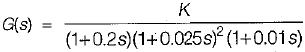Now, 20 log K = 100
∴ K = 105
Thus,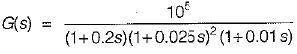QUESTION: 8

A unity feedback system has the open loop transfer function,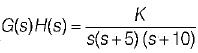For the given system to be marginally stable, the value of gain cross-over frequency would be

Solution:

For marginally stable system,
P.M = G.M = 0 and ωpc = wgc
Now, phase margin = (180° + ϕ)
Where,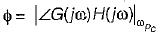Given, P.M = 0° (for marginally stable)
∴ 0º = 180° + ∠G(jω) H(jω)
or, ∠G(jω) H(jω) = -180°
Given,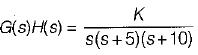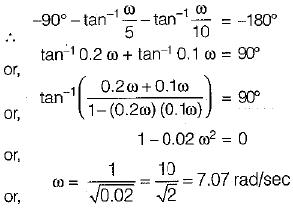Thus,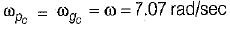QUESTION: 9

The transfer function of a lag-lead network is given by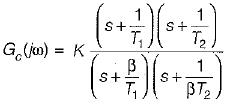The phase of Gc (jω) will become zero at

Solution:
QUESTION: 10

For the system shown below, what is the range of value of K for stability?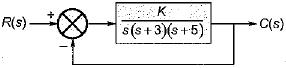Solution: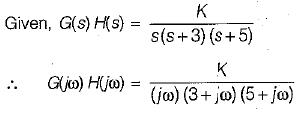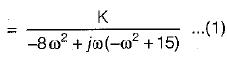The OLTF has no-poles in RH s-plane. Thus, for stability the -1 + j0 (critical point) point should not be encircled by Nyquist plot.
For finding the point of intersection of Nyquist piot with the negative reai axis.
Img [G(jω) H(jω)] = 0
or,  -ω2 + 15 = 0
or, ω = √15 rad /sec
Putting, ω = √15 rad/s in equation (1), we have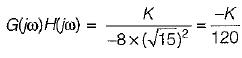For system to be stable,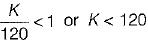So,
0 < K < 120 (for stability)

QUESTION: 11

Match List-I (Polar plot of systems) with List-ll (Transfer functions) and select the correct answer using the codes given below the lists:
List-I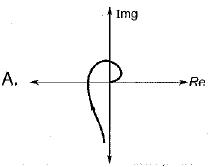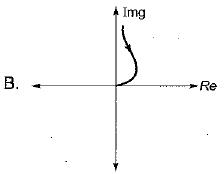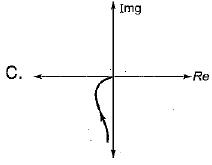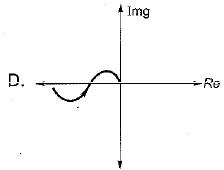List-II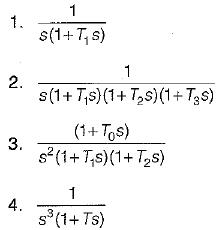Codes:
A B C D
(a) 3 1 2 4
(b) 2 4 1 3
(c) 2 1 4 3
(d) 1 2 3 4

Solution:
QUESTION: 12

Assertion (A): The Nyquist criterion represents a method of determining the location of the characteristic equation roots with respect to the left half and the right half of the s-plane.​
Reason (R): The Nyquist criterion does not give the exact location of the characteristic equation roots.

Solution:
QUESTION: 13

Which of the following is not true?

Solution:

BW ∝ ωn. Hence, option (d) is not correct. For a prototype second order system,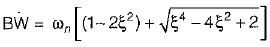Thus,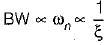QUESTION: 14

If the Nyquist plot cuts the negative real axis at a distance of 0.25, then the gain margin and phase margin of the system will be respectively

Solution:

Here,
|X| = 0.25 (Given)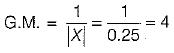Also, ϕ = -180°
∴  P.M. = 180 + ϕ
= 180°-180° = 0°

QUESTION: 15

Match List-I (Different values of damping ratio) with List-II (Values of resonant peaks) and select the correct answer using the codes given below the lists:
List-I
A. ξ = 0
B. ξ = 0.707
C. ξ = 0.85
D. ξ = 0.5

List-II
1. More than unity
2. Not defined
3. Unity
4. infinite
Codes:
A B C D
(a) 1 3 2 4
(b) 4 1 2 3
(c) 4 3 2 1
(d) 1 2 3 4

Solution:

Resonant peak is given by,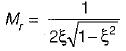• For ξ = 0, Mr = ∞
•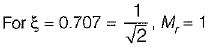•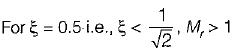•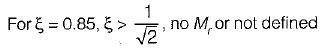In frequency domainanalysis,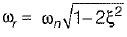Thus, for ωr to be real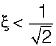Hence for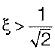there is no Mr or Mr is not defined.

QUESTION: 16

Assertion (A) : Gain margin alone is adequate to indicate relative stability when system parameters other than the loop gain are subject to variation.
Reason (R) : Gain margin is the amount of gain in dB that can be added to the loop before the closed-loop system becomes unstable.

Solution:

Gain margin alone is inadequate to indicate relative stability when system parameters other than the loop gain are subject to variation. Due to variation in some parameters, phase shift of the system can vary which may results instability. Hence, we must also consider the concept of phase margin for determining the relative stability of the system.

QUESTION: 17

Assertion (A): Phase margin is measured at the gain crossover frequency.
Reason (R): Gain crossover frequency can be easily determined from Bode plot than from the Nyquist plot.

Solution:
QUESTION: 18

If the magnitude of closed loop transfer function of a system is given by M, then the centre and radius of M-circle will be respectively given by:

Solution:
QUESTION: 19

The open loop transfer function of a unity feedback system is given by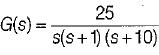The value of ωpc, is

Solution:

Given,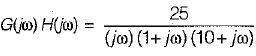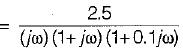∴ ϕ = ∠G(jω) H(jω)
= -90° - tan-1ω - tan-1 (0.1 ω)
At  ω = ωPc, ϕ = -180°, therefore
-180° = -90 ° - tan-1ω - tan-1 (0.1 ω)
or,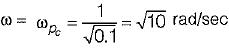= Phase crossover frequency

QUESTION: 20

If the gain of the open-loop system is increased to four times, the gain margin of the system

Solution: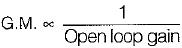∴ When open-loop gain becomes 4-times, GM will become one-fourth.